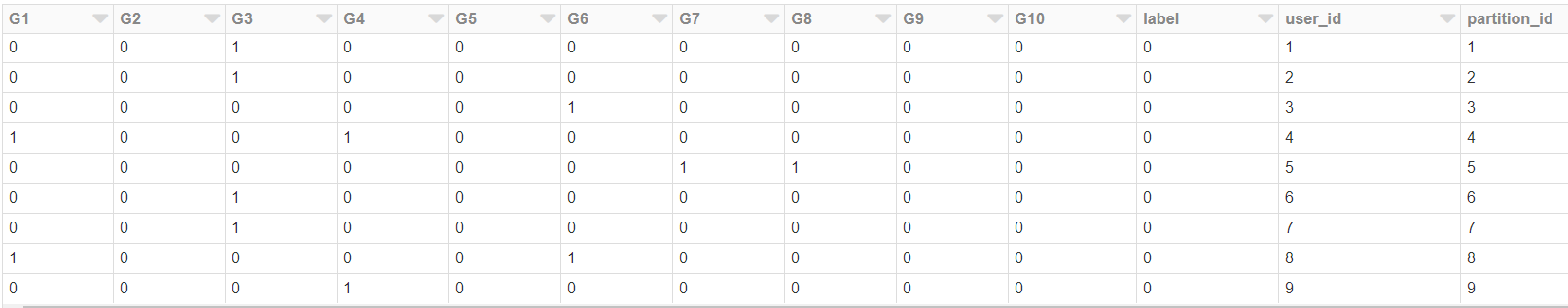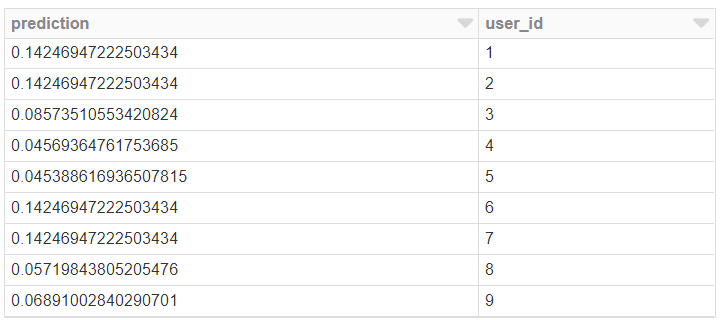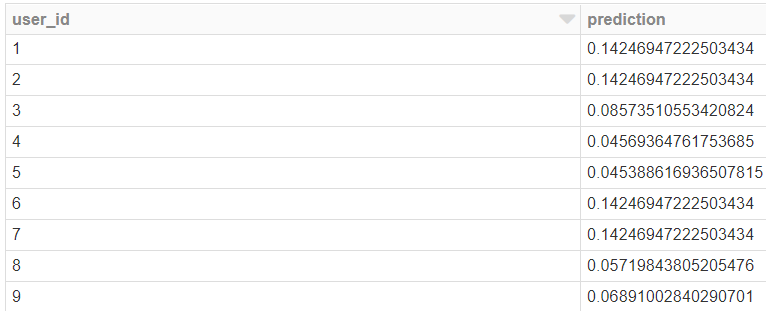# Making Python code run at massive scale in the cloudPySpark is a really powerful tool, because it enables writing Python code that can scale from a single machine to a large cluster.

While libraries such as MLlib provide good coverage of the standard tasks that a data scientists may want to perform in this environment, there’s a breadth of functionality provided by Python libraries that is not set up to work in this distributed environment. While libraries such as Koalas should make it easier to port Python libraries to PySpark, there’s still a gap between the corpus of libraries that developers want to apply in a scalable runtime and the set of libraries that support distributed execution. This post discusses how bridge this gap using the the functionality provided by Pandas UDFs in Spark 2.3+.

I encountered Pandas UDFs, because I needed a way of scaling up automated feature engineering for a project I developed at Zynga. We have dozens of games with diverse event taxonomies, and needed an automated approach for generating features for different models. The plan was to use the Featuretools library to perform this task, but the challenge we faced was that it worked only with Pandas on a single machine. Our use case required scaling up to a large cluster and we needed to run the Python library in a parallelized and distributed mode. I was able to present our approach for achieving this scale at Spark Summit 2019.

The approach we took was to first perform a task on the driver node in a Spark cluster using a sample of data, and then scale up to the full data set using Pandas UDFs to handle billions of records of data. We used this approach for our feature generation step in our modeling pipeline. This method can also be applied to different steps in a data science workflow, and can also be used in domains outside of data science. We provide a deep dive into our approach in the following post on Medium:

This post walks through an example where Pandas UDFs are used to scale up the model application step of a batch prediction pipeline, but the use case for UDFs are much more extensive than covered in this blog.

#### A Data Science Application

Pandas UDFs can be used in a variety of applications for data science, ranging from feature generation to statistical testing to distributed model application. However, this method for scaling up Python is not limited to data science, and can be applied to a wide variety of domains, as long as you can encode your data as a data frame and you can partition your task into subproblems. To demonstrate how Pandas UDFs can be used to scale up Python code, we’ll walk through an example where a batch process is used to create a likelihood to purchase model, first using a single machine and then a cluster to scale to potentially billions or records. The full source code for this post is available on github, and the libraries that we’ll use are pre-installed on the Databricks community edition.

The first step in our notebook is loading the libraries that we’ll use to perform distributed model application. We need Pandas to load our dataset and to implement the user-defined function, sklearn to build a classification model, and pyspark libraries for defining a UDF.

``````# load pandas, sklearn, and pyspark types and functions
import pandas as pd
from sklearn.linear_model import LogisticRegression
from pyspark.sql.functions import pandas_udf, PandasUDFType
from pyspark.sql.types import *
``````

sk_libs.py

Next, we’ll load a data set for building a classification model. In this code snippet, a CSV is eagerly fetched into memory using the Pandas `read_csv` function and then converted to a Spark dataframe. The code also appends a unique ID for each record and a partition ID that is used to distribute the data frame when using a PDF.

``````# load the CSV as a Spark data frame
"https://github.com/bgweber/Twitch/raw/master/Recommendations/games-expand.csv")
spark_df = spark.createDataFrame(pandas_df)

# assign a user ID and a partition ID using Spark SQL
spark_df.createOrReplaceTempView("spark_df")
spark_df = spark.sql("""
select *, user_id%10 as partition_id
from (
select *, row_number() over (order by rand()) as user_id
from spark_df
)
""")

# preview the results
display(spark_df)
``````

The output of this step is shown in the table below. The Spark dataframe is a collection of records, where each records specifies if a user has previously purchase a set of games in the catalog, the label specifies if the user purchased a new game release, and the user_id and parition_id fields are generated using the spark sql statement from the snippet above.We now have a Spark dataframe that we can use to perform modeling tasks. However, for this example we’ll focus on tasks that we can perform when pulling a sample of the data set to the driver node. When running the `toPandas()` command, the entire data frame is eagerly fetched into the memory of the driver node. This is fine for this example, since we’re working with a small data set. But it’s a best practice to sample your data set before using the toPandas function. Once we pull the data frame to the driver node, we can use sklearn to build a logistic regression model.

``````# train a model, but first, pull everything to the driver node
df = spark_df.toPandas().drop(['user_id', 'partition_id'], axis = 1)

y_train = df['label']
x_train = df.drop(['label'], axis=1)

# use logistic regression
model = LogisticRegression()
model.fit(x_train, y_train)
``````

sk_train.py

As long as your complete data set can fit into memory, you can use the single machine approach to model application shown below, to apply the sklearn model to a new data frame. However, if you need to score millions or billions of records, then this single machine approach may fail.

``````# pull all data to the driver node
sample_df = spark_df.toPandas()

# create a prediction for each user
ids = sample_df['user_id']
x_train = sample_df.drop(['label', 'user_id', 'partition_id'], axis=1)
pred = model.predict_proba(x_train)
result_df = pd.DataFrame({'user_id': ids, 'prediction': pred[:,1]})

# display the results
display(spark.createDataFrame(result_df))
``````

sk_driver.py

The outcome of this step is a data frame of user IDs and model predictions.In the last step in the notebook, we’ll use a Pandas UDF to scale the model application process. Instead of pulling the full dataset into memory on the driver node, we can use Pandas UDFs to distribute the dataset across a Spark cluster, and use pyarrow to translate between the spark and Pandas data frame representations. The result is the same as the code snippet above, but in this case the data frame is distributed across the worker nodes in the cluster, and the task is executed in parallel on the cluster.

``````# define a schema for the result set, the user ID and model prediction
schema = StructType([StructField('user_id', LongType(), True),
StructField('prediction', DoubleType(), True)])

# define the Pandas UDF
@pandas_udf(schema, PandasUDFType.GROUPED_MAP)
def apply_model(sample_pd):

# run the model on the partitioned data set
ids = sample_df['user_id']
x_train = sample_df.drop(['label', 'user_id', 'partition_id'], axis=1)
pred = model.predict_proba(x_train)

return pd.DataFrame({'user_id': ids, 'prediction': pred[:,1]})

# partition the data and run the UDF
results = spark_df.groupby('partition_id').apply(apply_model)
display(results)
``````

sk_pandas_udf.py

The result is the same as before, but the computation has now moved from the driver node to a cluster of worker nodes. The input and output of this process is a Spark dataframe, even though we’re using Pandas to perform a task within our UDF.For more details on setting up a Pandas UDF, check out my prior post on getting up and running with PySpark.

This was an introduction that showed how to move sklearn processing from the driver node in a Spark cluster to the worker nodes. I’ve also used this functionality to scale up the Featuretools library to work with billions of records and create hundreds of predictive models.

#### Conclusion

Pandas UDFs are a feature that enable Python code to run in a distributed environment, even if the library was developed for single node execution. Data scientist can benefit from this functionality when building scalable data pipelines, but many different domains can also benefit from this new functionality. I provided an example for batch model application and linked to a project using Pandas UDFs for automated feature generation. There’s many applications of UDFs that haven’t yet been explored and there’s a new scale of compute that is now available for Python developers.